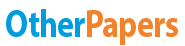# The Mole - Amount of a Substance

Essay by   •  January 7, 2019  •  Course Note  •  1,722 Words (7 Pages)  •  476 Views

## Essay Preview: The Mole - Amount of a Substance

Report this essay
Page 1 of 7

Moles

## The Mole

The ‘amount of a substance’ is often measured in grams. It could also be measured by counting the number of atoms, ions, or molecules present. However, because atoms weigh so little, it is more convenient to work on a larger scale and use moles.

I mole-

One mole of particles contains the same number of particles as there are atoms on exactly 12 of carbon 12.

600,000,000,000,000,000,000,000

This number is approximately 6 x 1023. The mass of 1 mole of a substance is equal to its Relative Mass in grams.

Basic Mole Equations

E.g.  Find the mass of:

1 mole of chlorine atoms                35.5 g

1 mole of water                                18 g

1 mole of sodium chloride                23 + 35.5 = 58.5 g

E.g.  What is the mass of 0.75 moles of carbon?

1 mole C        =        12 g

0.75 moles C        =        12 x 0.75 g

=        9 g

E.g.  How many moles of carbon are there in 10 g?

12 g contains 1 mole (as Ar C = 12)

12 g                =        1 mole

1 g                =         moles[pic 1]

10 g                =        10 x  moles[pic 2]

=        0.83 moles

E.g. How many moles of hydrogen atoms are there in 1 mole of ethanol (C2H6O)?

The formula of ethanol, C2H6O, tells us that 1 mole of ethanol contains:

2 moles of carbon atoms

6 moles of hydrogen atoms

1 mole of oxygen atoms

E.g.  Find the mass of chlorine in 10 g of magnesium chloride:

1st find how many moles of MgCl2 there are:

Atomic mass

95 g                =        1 mole MgCl2

1 g                 =         moles[pic 3]

10 g                =        10 x  moles[pic 4]

=        0.1053 moles of MgCl2

MgCl2                :        Cl

1                :        2

0.1053                :        0.2105 moles of Cl atoms

1 mole of Cl        =        35.5 g

0.2105 moles        =        35.5 x 0.2105 g

=        7.47 g of Cl in 10 g of MgCl2

## Stoichiometry

Stoichiometry or balancing of an equation can be found if the masses of the reactants and products in a particular reaction are known.

E.g.  Work out the stoichiometry for the reaction between 1.6 g of methane and 4.8 g of oxygen forming 3.6 g of water and one other product

 CH4 O2 H2O Mass 1.6 4.8 3.6 Mr 16 32 18 Moles 0.1 0.15 0.2 ÷ Smallest 1 1.5 2 Ratio 2 3 4

2CH4 + 3O2                               2CO +4H2O[pic 5]

## Further Moles and Equations

How to work out complicated equations

1. Write a balanced equation
2. Convert mass into moles
3. Use the equation
4. Convert moles into mass

E.g.  What mass of water vapour is formed when1.6 g of methane burns?

Step 1

Equation

CH4 + 2O2                   CO2 + 2H2O            [pic 6]

1.6 g                                      ?g

Step 2

Mass         Moles[pic 7]

16 g                =        1 mole

1 g                =         moles[pic 8]

...

...

Download as:   txt (4.3 Kb)   pdf (79.3 Kb)   docx (15.7 Kb)
Continue for 6 more pages »
Only available on OtherPapers.com
Citation Generator

(2019, 01). The Mole - Amount of a Substance. OtherPapers.com. Retrieved 01, 2019, from https://www.otherpapers.com/essay/The-Mole-Amount-of-a-Substance/65268.html

"The Mole - Amount of a Substance" OtherPapers.com. 01 2019. 2019. 01 2019 <https://www.otherpapers.com/essay/The-Mole-Amount-of-a-Substance/65268.html>.

"The Mole - Amount of a Substance." OtherPapers.com. OtherPapers.com, 01 2019. Web. 01 2019. <https://www.otherpapers.com/essay/The-Mole-Amount-of-a-Substance/65268.html>.

"The Mole - Amount of a Substance." OtherPapers.com. 01, 2019. Accessed 01, 2019. https://www.otherpapers.com/essay/The-Mole-Amount-of-a-Substance/65268.html.